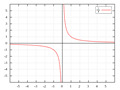# zero divide

what is zero divide. metatrader is givin zero divide error. I'm building the robot. wiping oneself
Files:

Kutluhan Yesilkaya:
what is zero divide. metatrader is givin zero divide error. I'm building the robot. wiping oneself
Surprisingly it's because your code is trying to calculate a division by zero, which is hardly possible.

https://en.wikipedia.org/wiki/Division_by_zero

In ordinary arithmetic, the expression (a/0) has no meaning, as there is no number which, when multiplied by 0, gives a (assuming a ≠ 0), and so division by zero is undefined

In computing, a program error  may result from an attempt to divide by zero.

You need to pre-check that any division in your algorithm is not by zero.Division by zero - Wikipedia
• en.wikipedia.org
The function y = 1/x. As x approaches 0 from the right, y approaches infinity. As x approaches 0 from the left, y approaches negative infinity. In mathematics, division by zero is division where the divisor (denominator) is zero. Such a division can be formally expressed as where a is the dividend (numerator). In ordinary arithmetic, the...

Kutluhan Yesilkaya :
what is  zero divide . metatrader is givin zero divide error. I'm building the robot. wiping oneself

Your code on line 161 contains a divide operation. You do not check your "divisor". Your "divisor" is zero.

While doing a division with a variable value you ALWAYS need to check if this value is not zero if you don't want your program to crash and restarting it manually...

Have you checked to make sure any indicators you are trying to use are loading/working correctly?
Reason: